# agricolaeplotr: a package for visualization of design of experiments

## Installation of the package

Use the following command to install the package from CRAN:

install.packages("agricolaeplotr")

# Usage of the package

This chapter shows the usage of the package and the underlying functions. As factorial experiments are omnipresent in all science and technology fields, a factorial ab-design will be used as an example. Although some parameters are worth for agriculture only, most other are useful for every user.

use the following command to load the package after installation. The two packages below agricolaeplotr are only needed for the examples.

library("agricolaeplotr")

library("ggplot2")
library("agricolae")

## Example: factorial ab design with complete randomization

First we need to create an design using agricolae package. All examples used in the package are directly taken from agricolae.

After creation of the object, everything is ready to plot a basic plot. It is also assumed, that the height and the width of each plot is set to 1. In agricultural designs, it is recommended to input the measures from a plot to have an idea about the dimensions needed to establish such a experiment in the field. Knowing the needed amount in meters or other units is important for machinery and management of the experiment.

Complete randomized designs do not have a factor like blocks, so the user is required to input a suitable number for columns and rows. The product of the number of rows and columns must be greater than the size of the experiment, to give the program the chance to place all plots.

Following figure shows the output of a basic design. The output is a standard ggplot2 design. This implies that the user can do all operations, that ggplot2 and other packages that uses ggplot2 functions, can be applied without having layer restrictions or too specialized layers that prevent other transformations. The user also might use plotly to create interactive visualizations of the designs. Useful for field demonstrations for all project stakeholders i.e. (scientists, farmers, funding agencies).

library(agricolae) # origin of the needed design object
trt<-c(3,2) # factorial 3x2
outdesign <- design.ab(trt, r=3, serie=2,design = 'crd')

head(outdesign$book,10) ## plots r A B ## 1 101 1 3 1 ## 2 102 1 2 1 ## 3 103 1 1 1 ## 4 104 1 3 2 ## 5 105 2 2 1 ## 6 106 2 3 1 ## 7 107 2 1 1 ## 8 108 2 3 2 ## 9 109 1 1 2 ## 10 110 1 2 2 plot_design.factorial_crd(outdesign,ncols=7,nrows=3, width = 1, height = 1)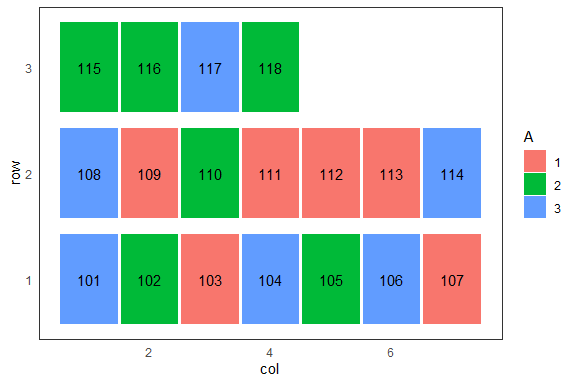# Change Axis and orientation of the design The user can change the order of both axis. Although it is a standard to enumerate plots from left to right, increasing from bottom, there is no restriction to enumerate in other ways. The following change reverts the y axis. Reverting y axis causes to have same orientation as ‘agricolae’ packages shows in some sketches. If the user wants to have same orientation, one must set reverse_y=TRUE. ## revert the y axis plot_design.factorial_crd(outdesign,ncols=6,nrows=3, width = 1, height = 1, reverse_y = TRUE)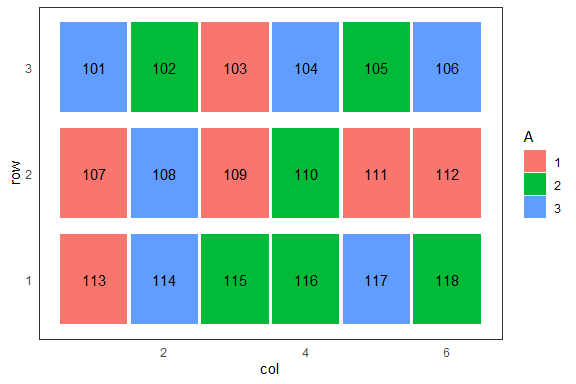## revert the x axis plot_design.factorial_crd(outdesign,ncols=6,nrows=3, width = 1, height = 1, reverse_x = TRUE)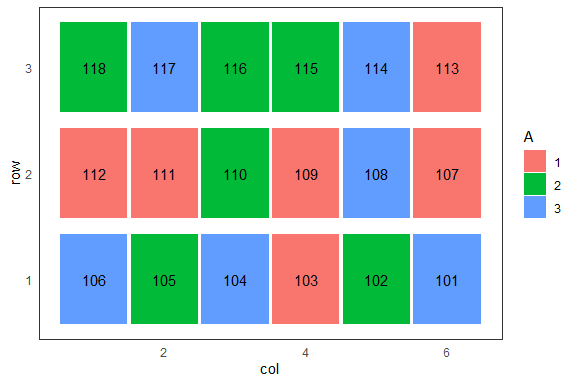## revert both axis plot_design.factorial_crd(outdesign,ncols=6,nrows=3, width = 1, height = 1, reverse_x = TRUE,reverse_y = TRUE)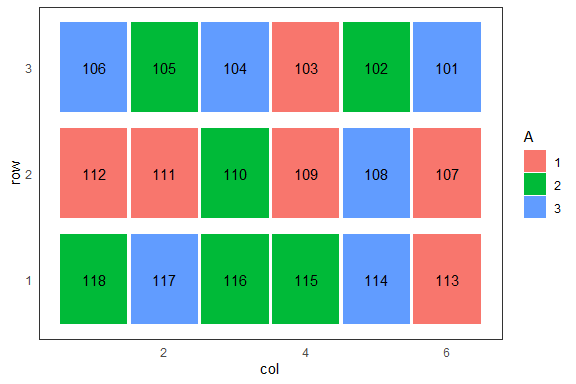## Changing width and height As described before, the user can decide the dimensions of each plot. The function assumes that each plot has same dimensions as the others. The dimensions are meant for a single plot, not for the entire field dimensions. plot_design.factorial_crd(outdesign,ncols=6,nrows=3, width = 1, height = 2.5 , reverse_x = FALSE,reverse_y = TRUE)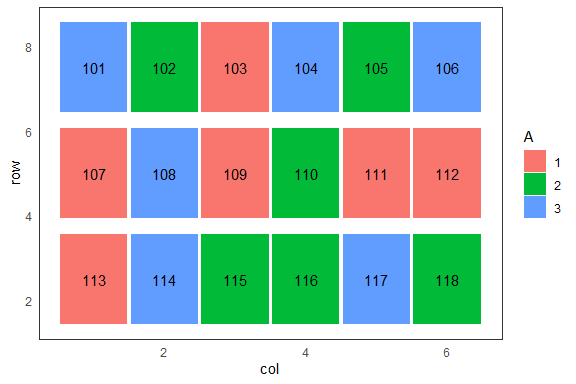## Changing space_width and space_height These parameters revert to the space of each plot. If both set to 1, there is no space between each plot. Zero indicates a 100 percent space between each plot, so no rectangle will be shown. Setting values greater one causes in overlaying plots, which are not possible in agricultural experimental design. Therefore the range of this parameter is between >0 and 1. plot_design.factorial_crd(outdesign,ncols=6,nrows=3, width = 1, height = 2.5 , reverse_x = FALSE,reverse_y = TRUE,space_width = 1,space_height = 1)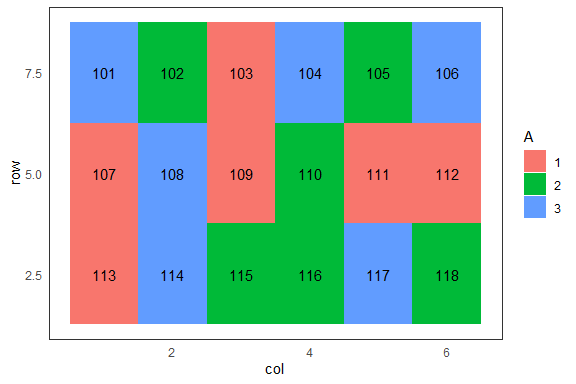This plot will show more space between each plot. If calculating the net area, the space parameter needs to be multiplied with the width and height respectively. plot_design.factorial_crd(outdesign,ncols=6,nrows=3, width = 1, height = 2.5 , reverse_x = FALSE,reverse_y = TRUE,space_width = 0.7,space_height = 0.8)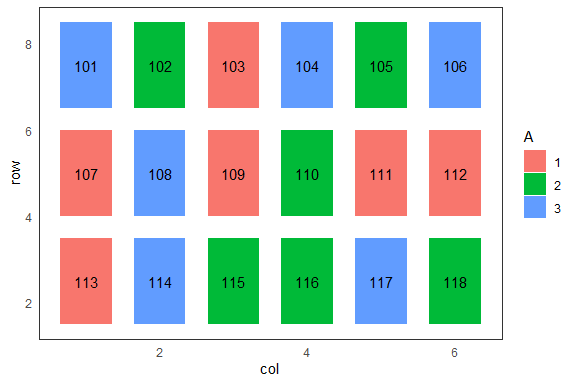## Change of label column The user can input a column name as a string, that must be in the columns of the design$book table. The input will be checked if it does so.

plot_design.factorial_crd(outdesign,ncols=6,nrows=3, width = 1, height = 2.5 , reverse_x = FALSE,reverse_y = TRUE, factor_name = "B")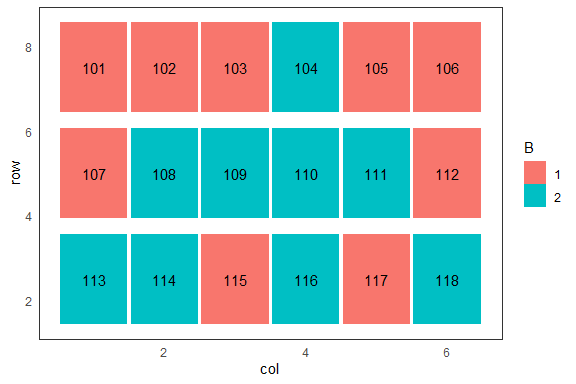The user can input a factor_name as a string, that must be in the columns of the design$book table. The input will be checked if it does so. This example shows how the selection of a different factor than the first one (default) will be performed for factorial experiments in complete randomized designs. set.seed(129984) trt<-c(3,2) # factorial 3x2 outdesign <- design.ab(trt, r=3, serie=2,design = 'crd') plot_design.factorial_crd(outdesign,ncols=3,nrows=6, width = 1, height = 2.5 , reverse_x = FALSE,reverse_y = TRUE, factor_name = "B")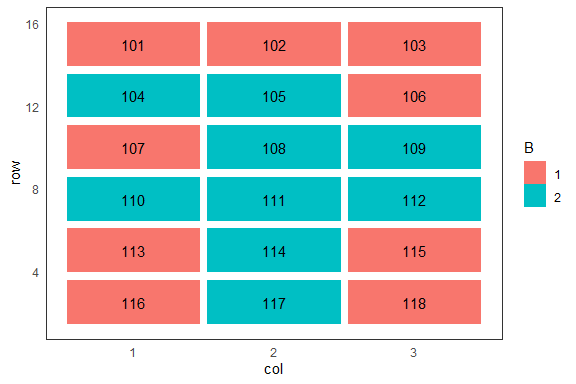## poster_theme This function adds changes to a ggplot, which are good for poster presentations. set.seed(129866478) trt<-c(3,2) # factorial 3x2 outdesign <- design.ab(trt, r=3, serie=2,design = 'crd') plot_design.factorial_crd(outdesign,ncols=3,nrows=6, width = 1, height = 2.5 , reverse_x = FALSE,reverse_y = TRUE, factor_name = "B") + theme_poster()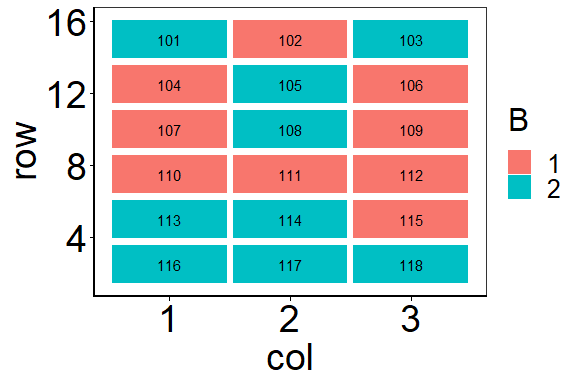## plotting continuous outcomes Plotting design maps like a heat map has some advantages. First, it shows some generals eyeballing trends in the data. Second, missing data are shown as a plot, therefore a technician can go to the experiment and retake samples, if needed. NA are plotted in gray. ## pres_theme This function adds changes to a ggplot, which are good for presentations in slideshows. set.seed(12986) trt<-c(3,2) # factorial 3x2 outdesign <- design.ab(trt, r=3, serie=2,design = 'crd') plot_design.factorial_crd(outdesign,ncols=3,nrows=6, width = 1, height = 2.5 , reverse_x = FALSE,reverse_y = TRUE, factor_name = "B") + theme_pres()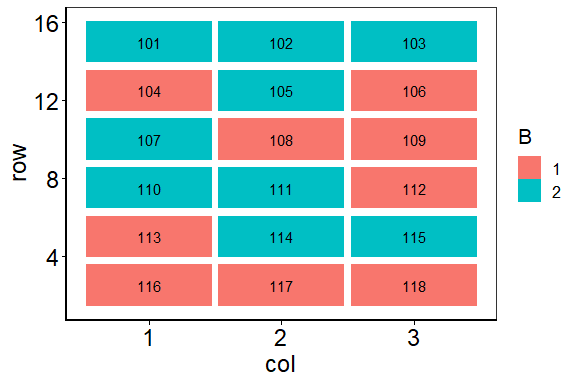## re-scaling colors The user can use a scale for factors. Sometimes useful if factors are in a gradient manner, for example nitrogen fertilizer application or amount of irrigation in Liter. trt<-c(3,2) # factorial 3x2 outdesign <- design.ab(trt, r=3, serie=2,design = 'crd') plot_design.factorial_crd(outdesign,ncols=3,nrows=6, width = 1, height = 2.5 , reverse_x = FALSE,reverse_y = TRUE, factor_name = "B") + theme_pres() + scale_fill_viridis_d()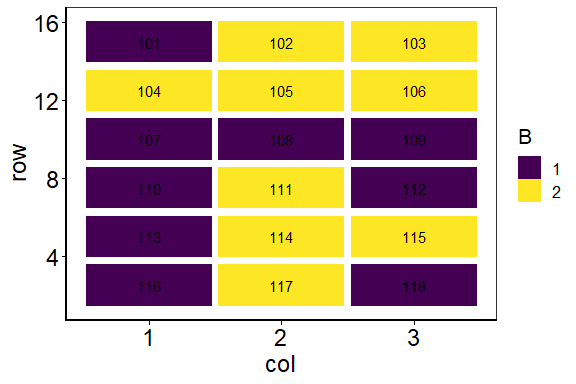## Plotting of exogenous variables In experiment analysis, a visualization of the results prior to analysis is an important step in assuring a high quality. identifying plots with missing data enables a re-sampling of the missing plots, so less numeric data imputation will be necessary. The following example shows, how to plot an exogenous variable. The variable is here the factor_name. We assume that we want to plot yield of a crop experiment, and use in addition a scaling parameter to recolor the fill. The code is the following: set.seed(23488833) trt <-c(3,2) outdesign<-design.ab(trt, serie=2, design="lsd",seed = 454555) length_table <- dim(outdesign$book) # length of the table

outdesign$book$yield <- sample(c(5:12,c(NA,NA,NA)), size = length_table, replace = TRUE)
plot_design.factorial_lsd(outdesign,factor_name = "yield") + scale_fill_viridis_c()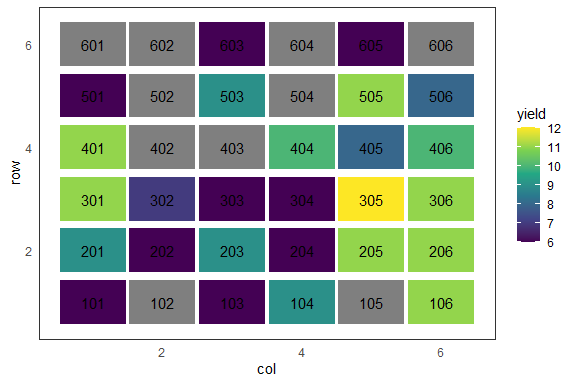The missing yields are easily to be seen.

## Plotting of exogenous variables from foreign tables

Sometimes, experiment data are in data frames, that must be merged with the outdesign object. Therefore, a second data frame will be merged with the outdesign and the merged data frame will be stored in the outdesign$book. set.seed(23488833) trt <-c(3,2) outdesign<-design.ab(trt, serie=2, design="lsd",seed = 454555) length_table <- dim(outdesign$book) # length of the table

yield <- sample(c(5:20,c(NA,NA,NA)), size = length_table, replace = TRUE)
df <- cbind(plots=outdesign$book$plots,yield)
head(df,10)
##       plots yield
##  [1,]   101    NA
##  [2,]   102    NA
##  [3,]   103     9
##  [4,]   104    13
##  [5,]   105    19
##  [6,]   106    19
##  [7,]   201    NA
##  [8,]   202     9
##  [9,]   203     6
## [10,]   204    11
outdesign$book <- merge(outdesign$book,df, by.x = "plots", by.y = "plots")
plot_design.factorial_lsd(outdesign,factor_name = "yield") + scale_fill_viridis_c()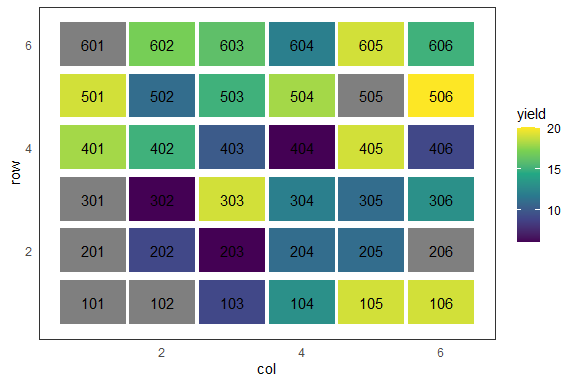## use your own design tables

It is also possible to use own generated experiments designs. However this comes with some caveats. As the functions are quite strict in parameter naming, the column names should match with those from agricolae. The design type and the applied design, sometimes referred as design type, needs to be set in a list in advance.

The following example provides a mock setup for an experiment. The second chunk of code shows how the list is set up and how the parameters should be named for a randomized complete block design in a factorial way. It is possible to plot measured outcomes from plots, as ggplot supports plotting continuous values.

set.seed(1298664)
plots <- as.factor(1:(8*6))
block <- as.factor((rep(1:6,each=8)))
A <- as.vector(replicate(8,sample(rep(1:2,times=3),6,replace=FALSE)))
outcome <- runif(48,20,100)
experiment <- cbind(plots,block,A,outcome)
experiment <- as.data.frame(experiment)
head(experiment)
##   plots block A  outcome
## 1     1     1 1 37.89540
## 2     2     1 2 61.40711
## 3     3     1 2 63.09927
## 4     4     1 1 98.15421
## 5     5     1 2 31.26676
## 6     6     1 1 90.85659
experiment_design <- list()
experiment_design$parameters$design<- "factorial"
experiment_design$parameters$applied <-  "rcbd"

experiment_design$book <- experiment head(experiment_design) ##$parameters
## $parameters$design
##  "factorial"
##
## $parameters$applied
##  "rcbd"
##
##
## \$book
##    plots block A  outcome
## 1      1     1 1 37.89540
## 2      2     1 2 61.40711
## 3      3     1 2 63.09927
## 4      4     1 1 98.15421
## 5      5     1 2 31.26676
## 6      6     1 1 90.85659
## 7      7     1 1 59.96641
## 8      8     1 2 55.55669
## 9      9     2 1 93.40734
## 10    10     2 1 81.13919
## 11    11     2 2 59.49315
## 12    12     2 2 66.98163
## 13    13     2 2 36.60583
## 14    14     2 1 65.60282
## 15    15     2 1 59.15911
## 16    16     2 1 99.62496
## 17    17     3 2 81.11360
## 18    18     3 2 45.91223
## 19    19     3 2 43.13358
## 20    20     3 1 54.39296
## 21    21     3 1 99.81043
## 22    22     3 2 64.92204
## 23    23     3 2 57.84001
## 24    24     3 1 68.44613
## 25    25     4 1 32.92130
## 26    26     4 1 51.88154
## 27    27     4 1 43.57497
## 28    28     4 2 42.05324
## 29    29     4 2 41.76551
## 30    30     4 2 28.24783
## 31    31     4 2 86.46124
## 32    32     4 1 90.25177
## 33    33     5 1 50.94946
## 34    34     5 2 23.45559
## 35    35     5 1 61.84104
## 36    36     5 2 60.42443
## 37    37     5 2 38.63727
## 38    38     5 2 74.36960
## 39    39     5 1 56.44705
## 40    40     5 1 91.73986
## 41    41     6 2 22.08535
## 42    42     6 1 35.80243
## 43    43     6 2 90.73155
## 44    44     6 2 43.10991
## 45    45     6 1 90.60870
## 46    46     6 1 89.13865
## 47    47     6 2 76.97044
## 48    48     6 1 62.76907
plot_design.factorial_rcbd(experiment_design,factor_name = "A")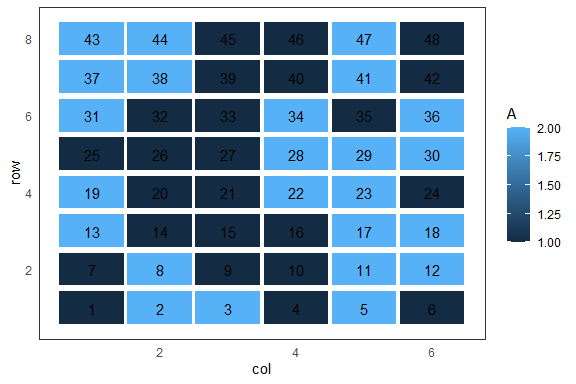plot_design.factorial_rcbd(experiment_design,factor_name = "outcome")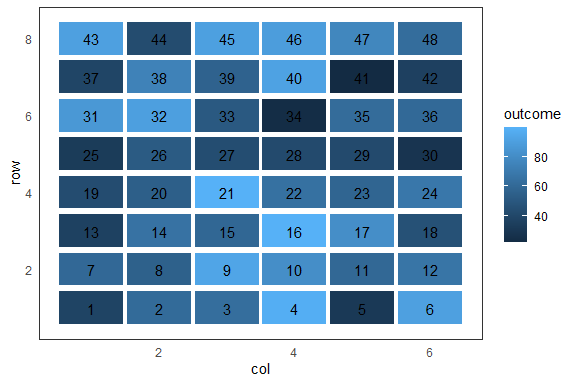## plot split plot designs

Split plots designs are designs that divide a main plot into two or more smaller, equal sized subplots. This leads to the definition of the dimensions. Te dimensions are meant for a single main plot, the super set of all belonging subplots. The three functions of this family are returning two plots. One for the main factor, a second one for the subplots. The dimension for the subplots are those from main plot, divided by the number of subplots, in width. To ensure only one plot is produced at the time, the user is required to choose a subplot design or an main plot design. To have both plots, the user has currently to run the function twice.

set.seed(1298664)
t1<-c('a','b','c','d','e',"f","g","h")
t2<-c("u",'v','w','x','y',"z")
outdesign2 <- design.split(trt1=t1, trt2=t2, r=r,serie = 2,
seed = 0, kinds = 'Super-Duper',
randomization=TRUE,first=TRUE,design = 'lsd')

plot_split_lsd(outdesign2,factor_name_1 = "t1",factor_name_2 = "t2",width = 2,height = 2, subplots = FALSE,labels = "plots")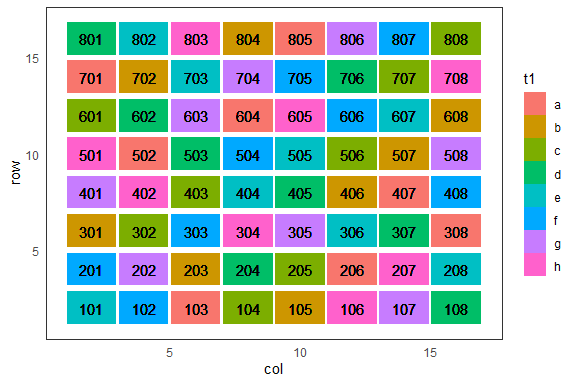plot_split_lsd(outdesign2,width = 2,height = 2, subplots = TRUE, labels = "splots", factor_name_1 = "t1", factor_name_2 = "t2")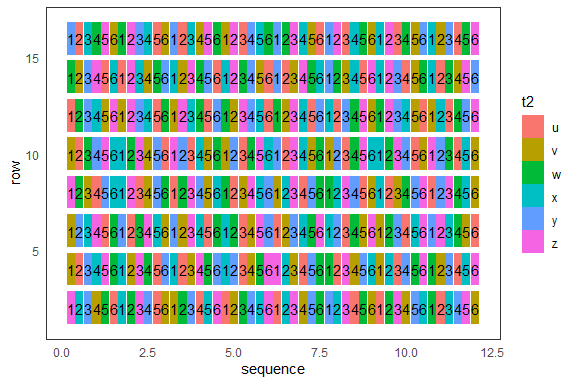set.seed(1298664)
t1<-c('a','b','c','d','e','f','g')
t2<-c('v','w','x','y','z')
r <- 4
outdesign2 <- design.split(trt1=t1, trt2=t2, r=r,
serie = 2, seed = 0, kinds = 'Super-Duper',
randomization=TRUE,first=TRUE,design = 'crd')
plot_split_crd(outdesign2,ncols = 5,nrows=6, subplots = FALSE,
factor_name_1 = "t1",factor_name_2 = "t2")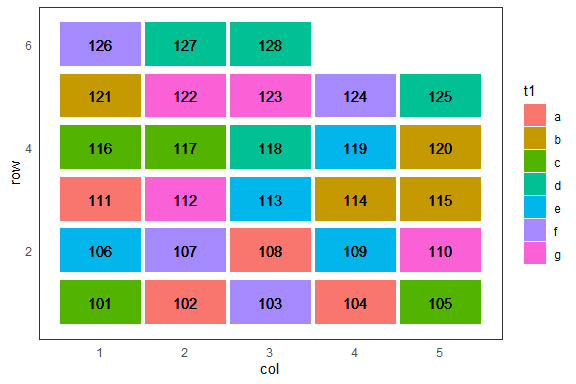plot_split_crd(outdesign2,ncols = 5,nrows=6, subplots = TRUE, labels="splots",factor_name_1 = "t1",factor_name_2 = "t2")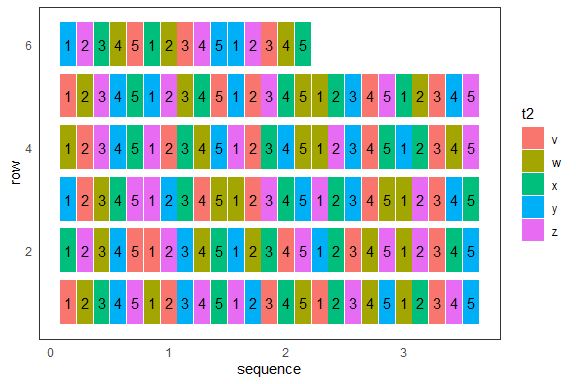set.seed(1298664)
T1<-c('a','b','c','d','e',"f","g")
T2<-c("we",'v','w','x','y','z',"d")
r = 3
outdesign2 <- design.split(trt1=T1, trt2=T2, r=r,serie = 2,
seed = 0, kinds = 'Super-Duper',randomization=TRUE,
first=TRUE,design = 'rcbd')

plot_split_rcbd(outdesign2,width = 5,height = 5,subplots = FALSE,
factor_name_1 = "T1",factor_name_2 = "T2")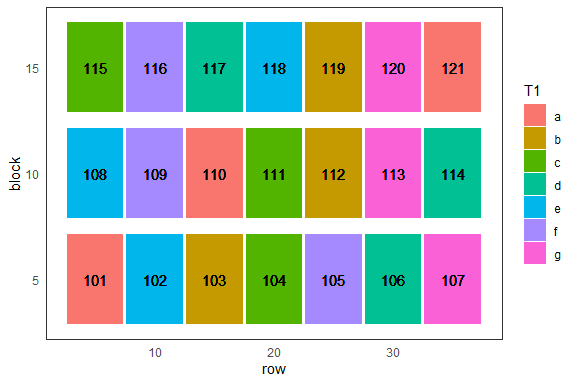plot_split_rcbd(outdesign2,width = 5,height = 5,labels = "splots",
factor_name_1 = "T1",factor_name_2 = "T2")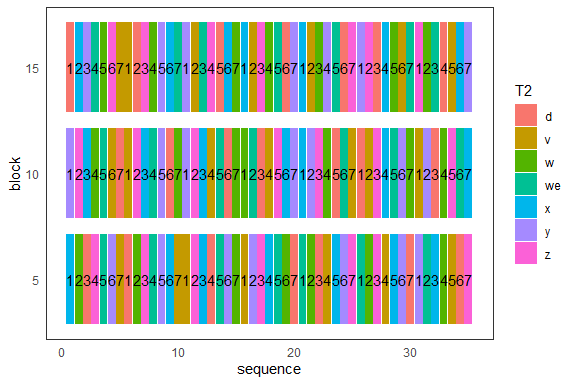## Referring to other packages

If the user can provide a table containing all necessary information about the coordinates of each plot, the user can choose the ‘desplot’ package.

There are two functions namely ‘ggdesplot’ and ‘ggdesplot’ from the ‘desplot’ package, that has some other features. However, the user cannot swap the x and y coordinates that easy as here and there is no way to adjust for height and length of a plot (preferable in meter). The user needs to provide x and y coordinates to be able to use ‘desplot’ and ‘ggdesplot’.

## Planed features for future versions

• match size of subplot to those to mainplots for all split plot designs
• create own function for user specific experiment designs with same API